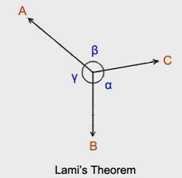Courses

# Introduction: Equilibrium of a Particle Mechanical Engineering Notes | EduRev

## Mechanical Engineering : Introduction: Equilibrium of a Particle Mechanical Engineering Notes | EduRev

The document Introduction: Equilibrium of a Particle Mechanical Engineering Notes | EduRev is a part of the Mechanical Engineering Course Engineering Mechanics - Notes, Videos, MCQs & PPTs.
All you need of Mechanical Engineering at this link: Mechanical Engineering

Equilibrium of Particle and Body

Equilibrium of a Particle When the resultant of all forces acting on a particle is zero, the particle is said to be in equilibrium.

A particle which is acted upon two forces.

Newton’s First Law:  If the resultant force on a particle is zero, the particle will remain at rest or will continue at constant speed in a straight line.

EQUILIBRIUM   A particle is in equilibrium if it is at rest if originally at rest or has a constant velocity if originally in motion. The term equilibrium or static equilibrium is used to describe an object at rest. To maintain equilibrium it is necessary to satisfy Newton’s first law of motion, which requires the resultant force acting on particle to be equal to zero. That is ∑F = 0 A Where ∑F = Sum of all the forces acting on the particle which is necessary condition for equilibrium. This follows from Newton’s second law of motion, which can be written as ∑F = ma. Put in equation A   ma = 0 Therefore the particle acceleration a = 0. Consequently the particle indeed moves with constant velocity or at rest.

Methods for the Equilibrium of Forces: There are many methods of finding the equilibrium but the following are important 1. Analytical Method 2. Graphical Method 1. Analytical method for the equilibrium of forces.The equilibrium of forces may be studied analytically by Lami’s theorem as discussed under

Lami’s Theorem: It states, “If there are three forces acting at a point be in equilibrium then each force is proportional to the sine of the angle between the other two forces”. Let three force F1, F2 and F3 acting at a point and the opposite angles to three forces are γ , β, and α as shown in figure

Mathematically             F1/Sin β  =  F2/Sin γ  =  F3 β/Sin αOffer running on EduRev: Apply code STAYHOME200 to get INR 200 off on our premium plan EduRev Infinity!

,

,

,

,

,

,

,

,

,

,

,

,

,

,

,

,

,

,

,

,

,

;